## Polynomial Root

If the Coefficients of the Polynomial(1)

are specified to be Integers, then integral roots must have a Numerator which is a factor of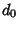and a Denominator which is a factor of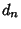(with either sign possible). This is known as the Polynomial Remainder Theorem.

Let the Roots of the polynomial(2)

be denoted,, ...,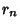. Then Newton's Relations are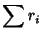(3)(4)(5)

These can be derived by writing(6)

expanding, and then comparing the coefficients with (2).

Any Polynomial can be numerically factored, although different Algorithms have different strengths and weaknesses.

If there are no Negative Roots of a Polynomial (as can be determined by Descartes' Sign Rule), then the Greatest Lower Bound is 0. Otherwise, write out the Coefficients, let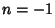, and compute the next line. Now, if any Coefficients are 0, set them to minus the sign of the next higher Coefficient, starting with the second highest order Coefficient. If all the signs alternate,is the greatest lower bound. If not, then subtract 1 from, and compute another line. For example, consider the Polynomial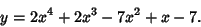(7)

Performing the above Algorithm then gives

 0 2 212 08-- 28272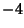5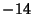35

so the greatest lower bound is.

If there are no Positive Roots of a Polynomial (as can be determined by Descartes' Sign Rule), the Least Upper Bound is 0. Otherwise, write out the Coefficients of the Polynomials, including zeros as necessary. Let. On the line below, write the highest order Coefficient. Starting with the second-highest Coefficient, addtimes the number just written to the original second Coefficient, and write it below the second Coefficient. Continue through order zero. If all the Coefficients are Nonnegative, the least upper bound is. If not, add one toand repeat the process again. For example, take the Polynomial(8)

Performing the above Algorithm gives

 0 211 2 1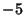2 2 33 2 5 8 25 68

so the Least Upper Bound is 3.

See also Bairstow's Method, Descartes' Sign Rule, Jenkins-Traub Method, Laguerre's Method, Lehmer-Schur Method, Maehly's Procedure, Muller's Method, Root, Zassenhaus-Berlekamp Algorithm